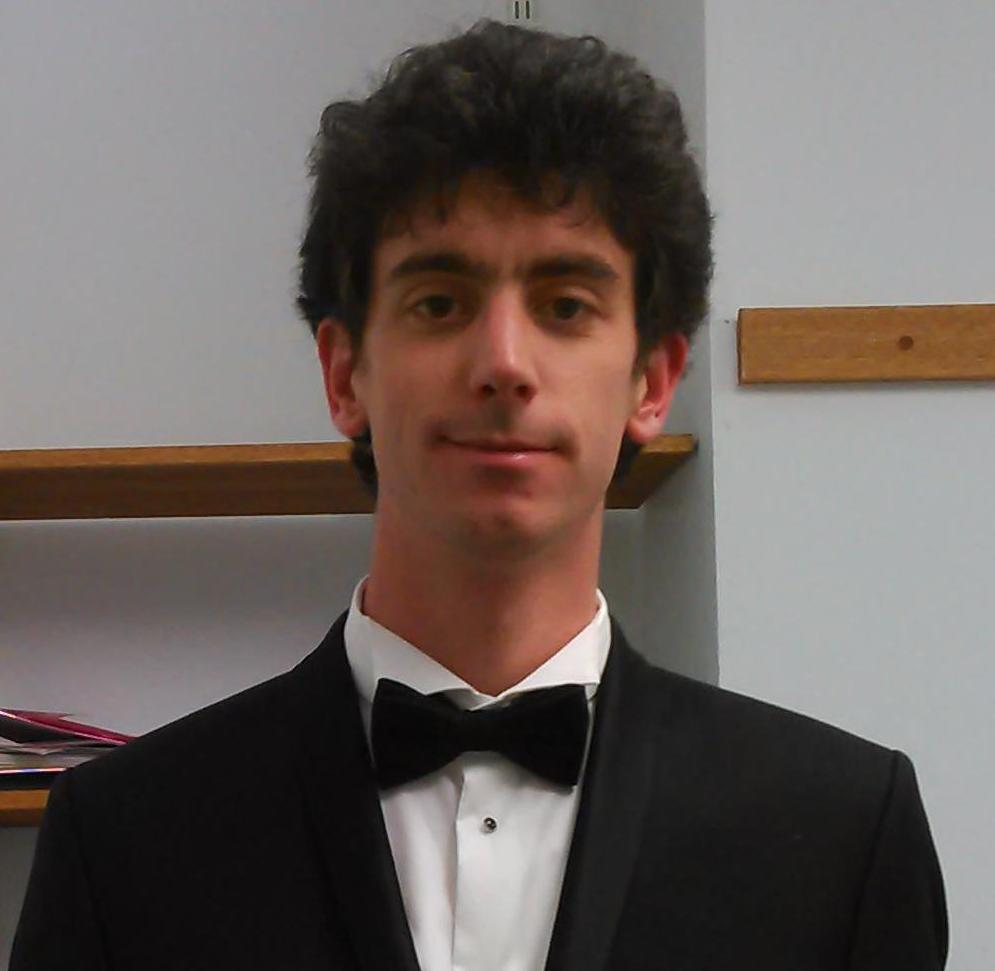# LSV Seminar

The LSV seminar takes place on Tuesday at 11:00 AM. The usual location is the conference room at Pavillon des Jardins (venue). If you wish to be informed by e-mail about upcoming seminars, please contact Stéphane Le Roux and Matthias Fuegger.

The seminar is open to public and does not require any form of registration.

## Past Seminars

### Point to point controllability in linear time invariant systemsDate
Tuesday, December 05 2017 at 11:00AM
Place
Salle de Conférence (Pavillon des Jardins)
Speaker
Amaury Pouly (MPI-SWS Saarbrücken)

We revisit a classical problem of control theory from the pint of view of computability. Consider a discrete time linear time invariant (LTI) system x(n+1)=Ax(n)+Bu(n) where x(n) is a vector of R^n, A and B are matrices and u(n) is an external input. Given x(0) and y, we want to know if there is a sequence of inputs u(0),..., u(k) such that x(k+1)=y. This problems models physical systems where x(n) is the state and u(n) is the input controlled the a user or a computer. We are therefore trying to steer the system to achieve some statereach some state y. In this talk, we will focus on the nontrivial case where the input u(n) is restricted to some convex state. Surprisingly, the problem becomes hard in general but we give positive results in some cases.